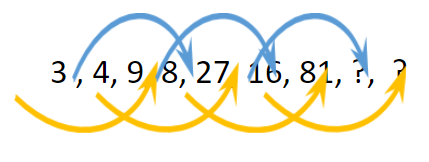#####What will you learn in this article?
• What are number series?
• Different types of number series
• Shortcuts for identifying the pattern of a series.
#####ExamConnect

In this topic, we will be given a series of numbers that follow a fixed pattern, and our job is to figure out the pattern used to make the series and find out the answer. Numeric Series is one of the most common topics in the logical reasoning section and is seen across all the different examinations. It not only tests the pattern recognition abilities of the examinee but also his/her presence of mind and analytical skills.

### Number Series: Definition and Types

As the name suggests, the series is made up of numbers that are arranged according to a specific pattern. There are two types of number series, which are as follows:

#### 1. Perfect sequence series

In this type of series, there is a single unique pattern that is followed throughout the series, it can be in the form of squares, cubes, addition, subtraction, multiplication, etc.

Example Question: Find the missing term for the series: 2, 4, 6, 8, ?

Solution: As we can see, the pattern followed in the above series is that:

2 is added in the previous number to get the next number i.e.

So the missing number in the series would be 10.

Example Question: Find the missing term: 4, 12, 36, 108, 324, ?

Solution: As we can see that this series is a Geometric progression with the common multiple ’3’.

So the missing term would be 324 × 3 = 972.

Example Question: 2, 3, 5, 7, 11, ?

Solution: Clearly, the series consists of prime numbers (numbers only having 2 factors), so the next prime number would be 13.

#### 2. Multiple sequence series

In this type of series, there are multiple unique patterns which are followed the series. The numbers following different patterns are independent of each other.

Example Question: Find the missing term for the series: 2, 3, 7, 6, 12, 9, 17, ?, ?

Solution: As we can see, that the above series as a whole does not have any specific pattern, this is because this series is a mixture of 2 distinct series that are stitched together as a single series.

So the above is a combination of the following series:

Hence, the missing terms are 12 and 22, respectively.EazyTip: Whenever you see a repeated number in a series or a series without any distinct ascending or descending order, then chances are it is a multiple pattern series.

Example Question: Find the missing term for the series: 3, 4, 9, 8, 27, 16, 81, ?, ?

Solution: As we can see, that the above series as a whole does not have any specific pattern, this is because this series is a mixture of 2 distinct series that are stitched together as a single series.So the above is a combination of the following series:

1. Multiple of 3 i.e. 3, 9, 27, 81, 243
2. Multiplying by 2 i.e. 4, 8, 16, 32

The missing terms are thus 32 and 243, respectively.

#### 3. Mixed number series

In this type of series, there is no single unique pattern which is followed across the series, rather the pattern followed keeps on changing or we can say that the pattern itself follows a unique pattern. Read the example given below for better understanding

Example Question: Identify the wrong number in the series: 5, 8, 17, 25, 37, 48, 65, 80.

Solution: As we can see, the series is not a perfect sequence series as there is no single pattern that governs the whole series, rather there are multiple patterns which themselves are following a pattern.

Like after simplifying the given series we get:

22 +1, 32 -1, 42 +1, 52 -1, 62 +1, 72 -1, 82 +1, 92 -1

So by following the pattern, we can see that 25 is the wrong number in the series.

Common mistakes: Students tend to over evaluate the patterns used in a series and, hence they get misdirected towards the wrong answer. It is recommended to remain calm and start analyzing the series from the ground up.EazyTip: Always start your analysis by checking whether the series consists of unique numbers such as prime numbers, then check the difference between consecutive numbers and finally check whether the series follows a single pattern system or multiple pattern system.